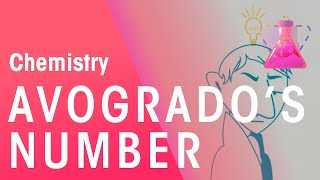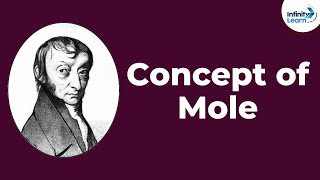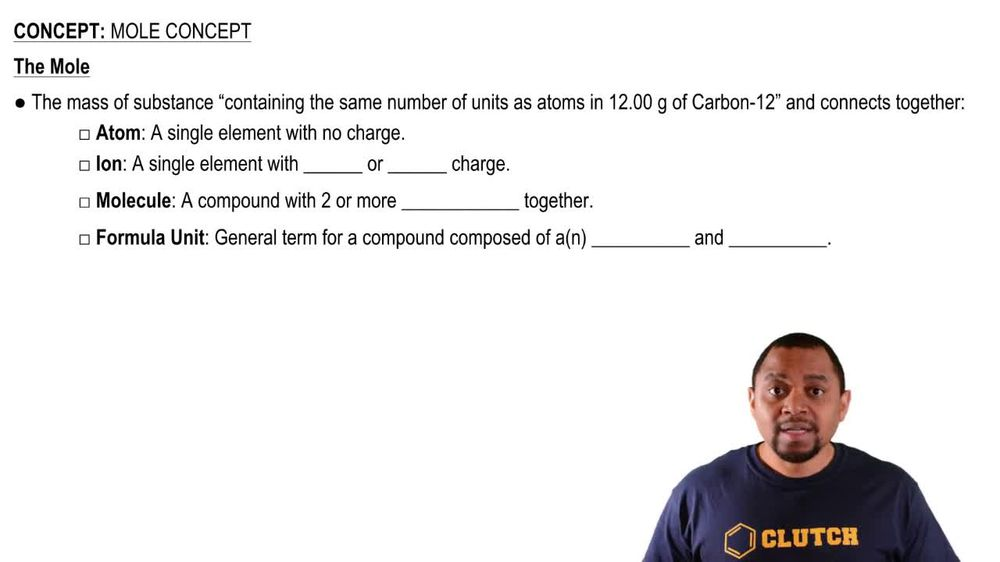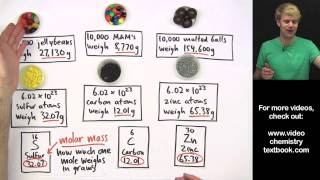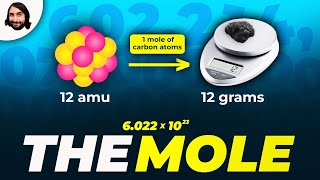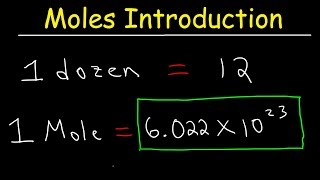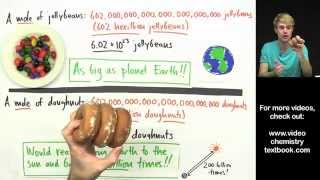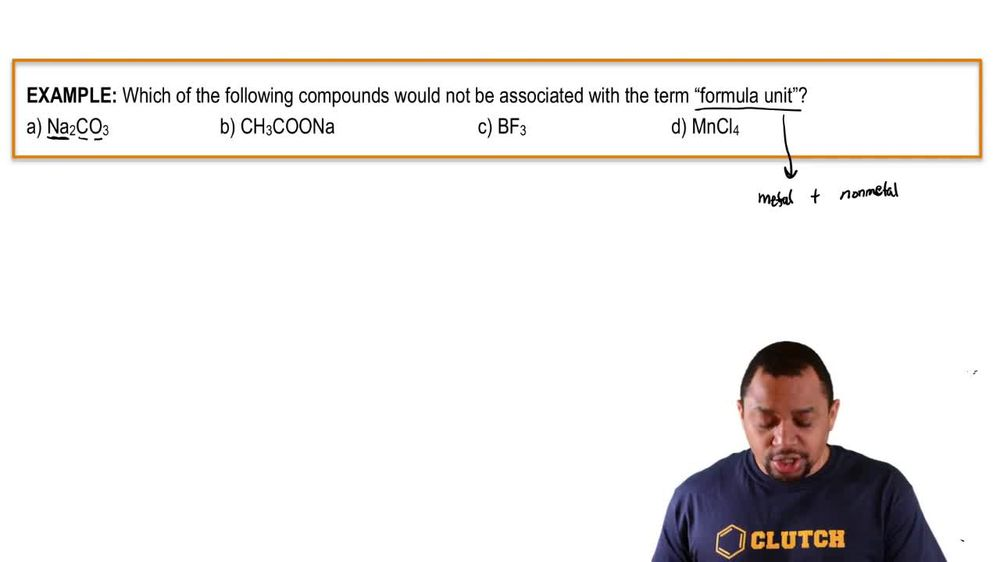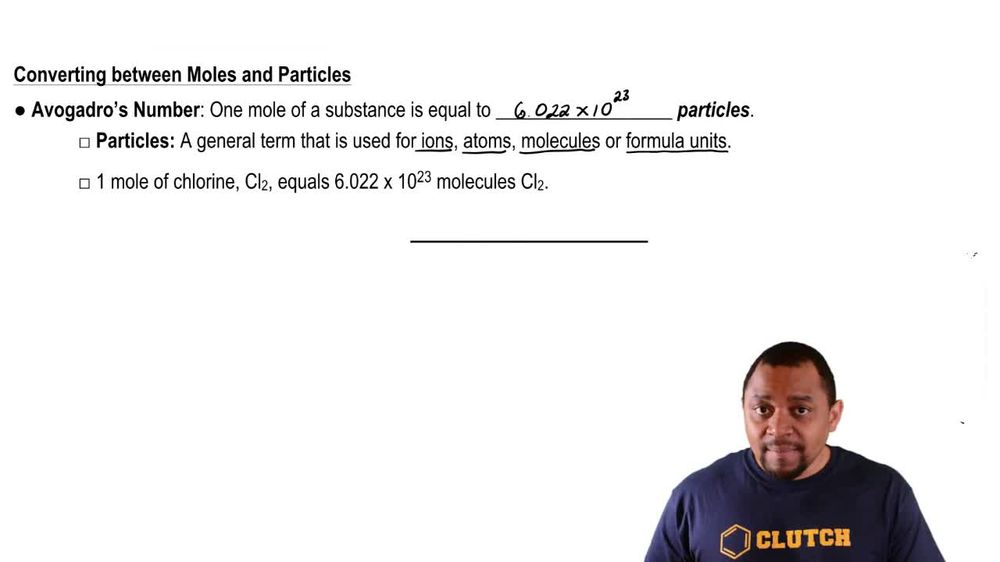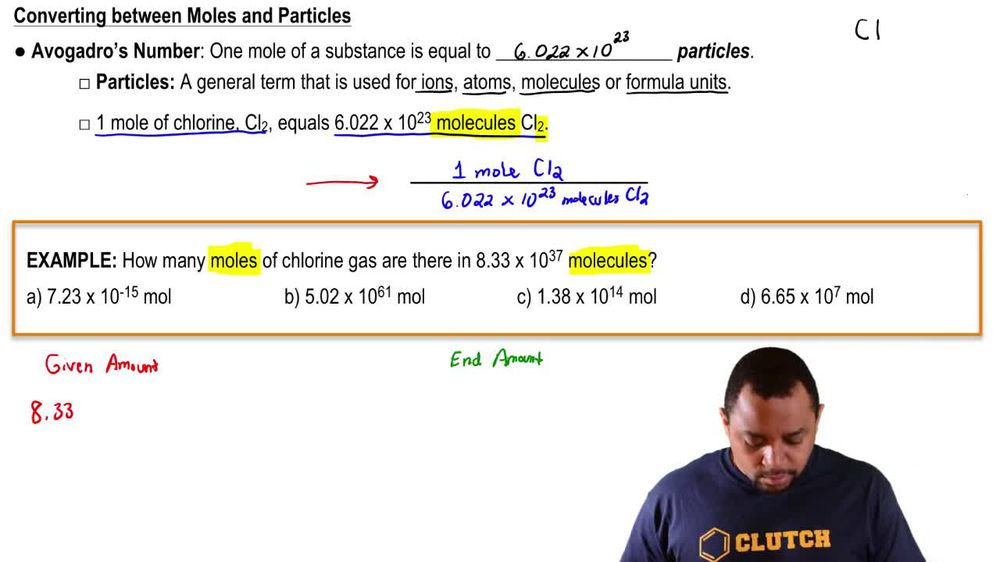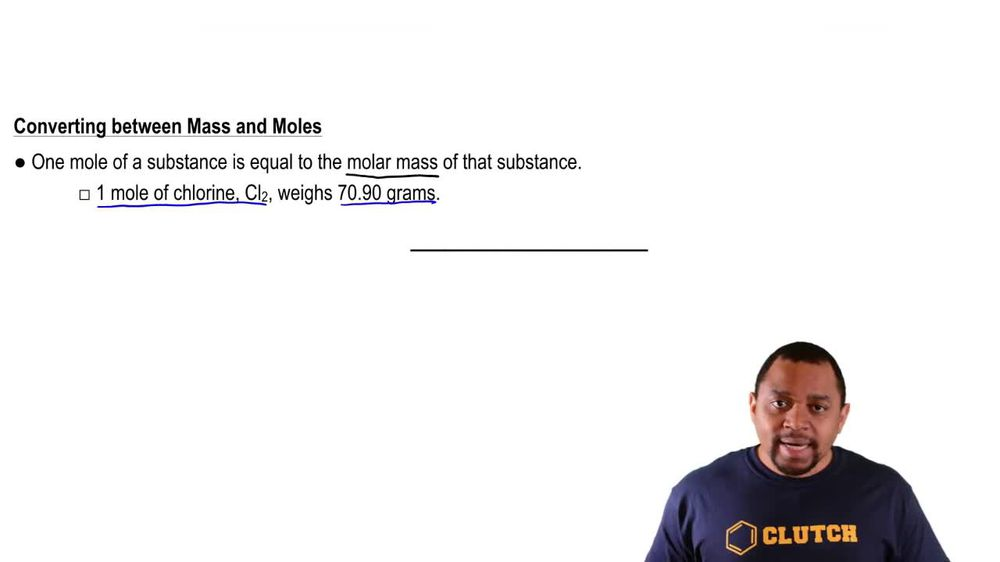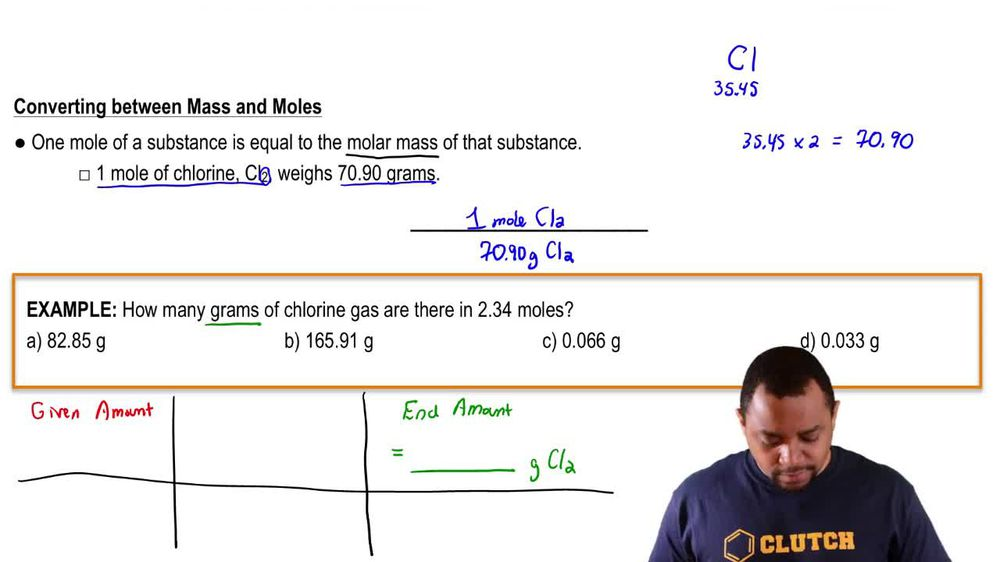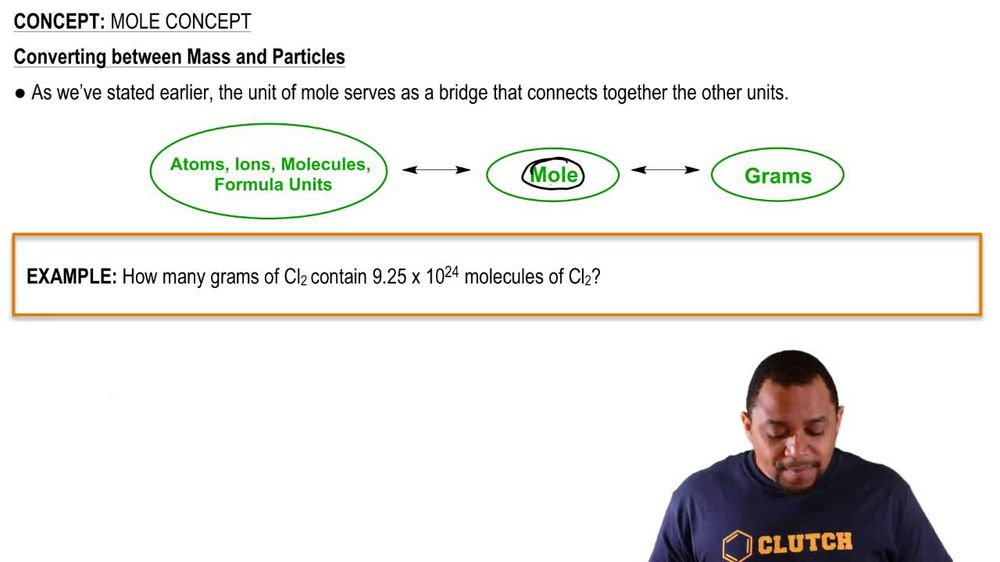Start typing, then use the up and down arrows to select an option from the list.
1. 2. Atoms & Elements2. Mole Concept
Problem

# How many molecules are in each sample? b. 389 g CBr4

Relevant Solution2m
Play a video:
Hi everyone today. We have a question asking us to assume that a tablet only contains paracetamol C eight H nine N 02. And to calculate the number of paracetamol molecules in a 500 mg tablet. So we're going to start out with our 500 mg of paracetamol And then we want to change two g. So we're going to multiply By 10 to the negative 3rd g over one mg and then we are going to multiply by one mole of paracetamol, divided by The molar mass of Paracetamol, which is 151.16 g. And then we're going to multiply by avocados number 6.02, 2 times 10 to the 23rd molecules over one mole of paracetamol. So our milligrams of paracetamol are canceling out. Our grams of paracetamol are canceling out are moles of paracetamol are canceling out, leaving us with molecules And that equals 1.99 Times 10 to the 21st molecules of paracetamol. And that is our final answer. Thank you for watching. Bye.